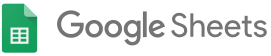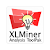Check out the store for many more# XLMiner Analysis ToolPak

(140)
offered by Frontline Systems Inc.160,645 users

## OverviewStatistical analysis in Google Sheets, with functions matching the Analysis ToolPak in desktop Excel.
```With the XLMiner Analysis ToolPak Add-on, you can easily perform statistical analyses of the same types available in the Analysis ToolPak add-in that has been part of Microsoft Excel for over 20 years, and has been featured in large numbers of textbooks, videos, statistics courses and the like.

The add-on provides all 19 interactive functions included in the Excel Analysis ToolPak, plus a 20th function often requested by users, logistic regression.  Sidebar input fields and output on the spreadsheet will be very familiar to Excel Analysis ToolPak users.

The 20 functions are:
ANOVA: Single Factor
ANOVA: Two-Factor with Replication
ANOVA: Two-Factor without Replication
Correlation
Covariance
Descriptive Statistics
Exponential Smoothing
F-Test Two-Sample for Variances
Fourier Analysis
Histogram
Linear Regression
Logistic Regression
Moving Average
Random Number Generation
Rank and Percentile
Sampling
t-Test: Paired Two-Sample for Means
t-Test: Two-Sample Assuming Equal Variances
t-Test: Two-Sample Assuming Unequal Variances
z-Test: Paired Two-Sample for Means

Results will generally match the Excel Analysis ToolPak, as seen in many textbooks, screen images and videos.  The add-on's Random Number Generation function uses the Mersenne Twister algorithm.
```

## Details

Version: 17
Updated: November 7, 2017
Language: English (United States)